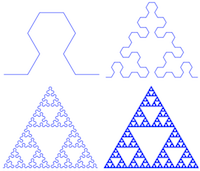# Sonic L-SystemsIn 1968, Aristid Lindenmayer introduced "L-systems" to the world. Basically, this is an iterative rewriting system of rules that operates on a string beginning with an "axiom" or initial string.

Using turtle graphics it is possible to draw all manner of fractal "pathological curves" with this technique.

Being a music nerd, I thought, "Why not make this play notes instead of draw lines?" And so the program [https://github.com/ology/Music/blob/master/lindenmayer-midi lindenmayer-midi] was born.

The program is short but has a few parts. The first is the preamble that says we are a perl program and that we will be fooling with MIDI things:

``````#!/usr/bin/env perl
use strict;
use warnings;

use MIDI::Util;
``````

Next, the program takes arguments from the command-line user:

``````my \$rule       = shift || 2,
my \$iterations = shift || 4;
my \$string     = shift || 'F';
my \$distance   = shift || 'qn';
my \$theta      = shift || 1;
``````

These variables specify the rule to use (shown below), the number of iterations to perform, the initial string (axiom), the "distance" – a musical duration like the quarter note, and theta – the amount to increase/decrease the current note value by.

Next up is to define the actual re-write rules to use:

``````my %rules = (
...
5 => {
F => 'G-F-G',
G => 'F+G+F',
},
...
);
``````

The program then initializes a MIDI score and sets the initial note to middle C (MIDI note 60):

``````my \$score = MIDIUtil::setup_midi( patch => 0, bpm => 300 );
my \$note = 60;
``````

Ok. Now for the meat of the program – a dispatch table of MIDI and note events, re-writing the string according to the given rules, and finally translating each string symbol into a dispatched command:

``````my %translate = (
'f' => sub { \$score->r(\$distance) },
'F' => sub { \$score->n( \$distance, \$note ) },
'G' => sub { \$score->n( \$distance, \$note ) },
'-' => sub { \$note -= \$theta },
'+' => sub { \$note += \$theta },
);

for ( 1 .. \$iterations ) {
\$string =~ s/(.)/defined(\$rules{\$rule}{\$1}) ? \$rules{\$rule}{\$1} : \$1/eg;
}
warn "\$string\n";

for my \$command ( split //, \$string ) {
\$translate{\$command}->() if exists \$translate{\$command};
}
``````

Lastly, the program writes the MIDI file that was created.

``````\$score->write_score( \$0 . '.mid' );
``````

Here are some examples. They are decidedly not music; more like Metroid on crack.

MIDI files: Sierpinski and Koch-islands-and-lakes. And here is an MP3 rendering of the former:

(On YouTube: , , , )

Not the easiest to dance to…